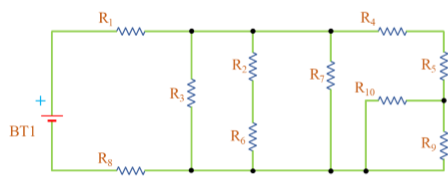Find the currents and voltages for the big circuit in the figure below. BT1 is a normal battery....

Question:

Find the currents and voltages for the big circuit in the figure below. BT1 is a normal battery.{eq}\begin{array}{|c|c|c|c|c|c|c|c|c|c|c|} \hline V_{bat} & R_1 & R_2 & R_3 & R_4 & R_5 & R_6 & R_7 & R_8 & R_9 & R_{10} \\ \hline 24 \, V & 5 \, \Omega & 12 \, \Omega & 4 \, \Omega & 4 \, \Omega & 6 \, \Omega & 16 \, \Omega & 8 \, \Omega & 5 \, \Omega & 2 \, \Omega & 4 \, \Omega\\ \hline \end{array} {/eq}

Ohm's Law in Parallel Circuits:

Kirchoff's Current Law can be time-consuming when there are many parallel paths in a circuit. We currents are hard to track in the parallel branches. To solve the currents, we use Ohm's Law since the parallel branches have the same potential differences across the branches.

Become a Study.com member to unlock this answer!

Let's determine the source current by Ohm's Law. To do that, let's find the equivalent resistance R of the circuit. For that, let's use the symbol || ...Parallel Circuits: Calculating Currents with Ohm's Law

from

Chapter 9 / Lesson 6
2.6K

Understand Kirchhoff's law and Ohm's law for parallel circuits, and see how to find current in a parallel circuit using the parallel circuit current formula.